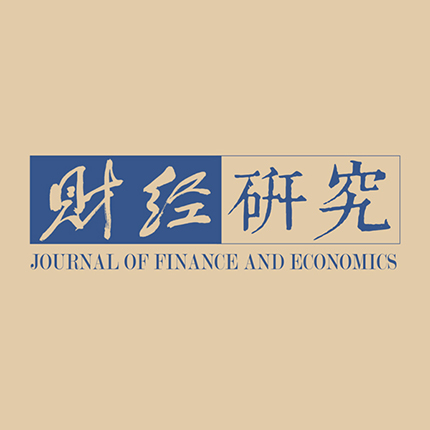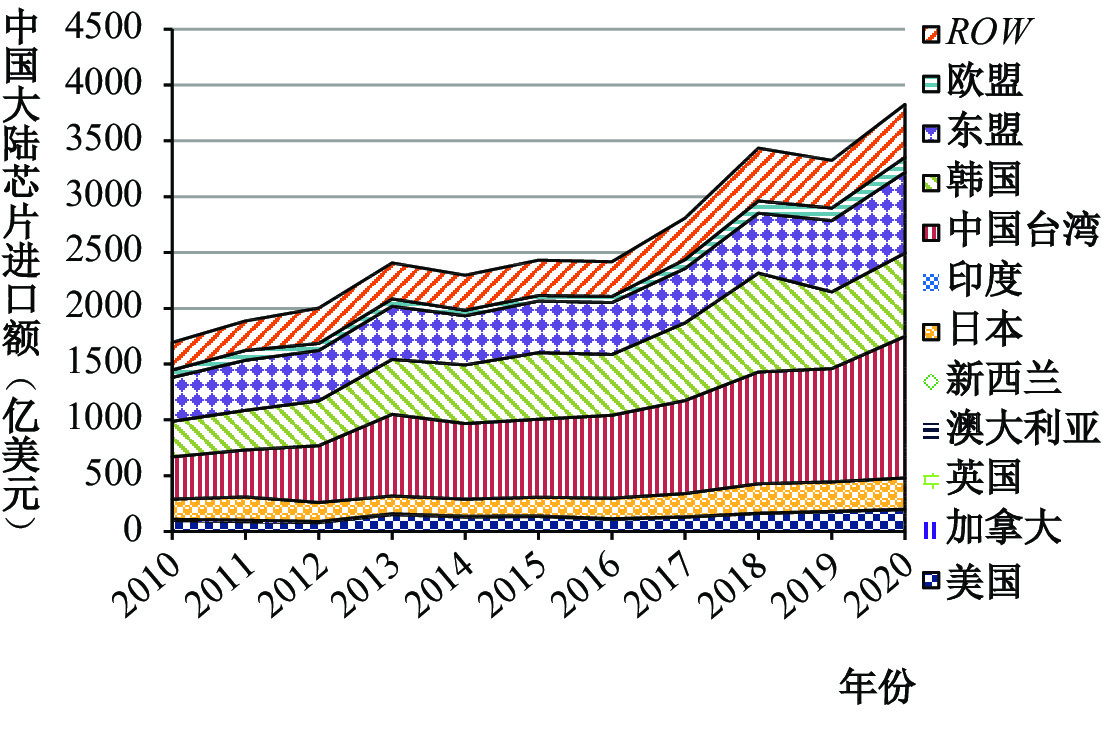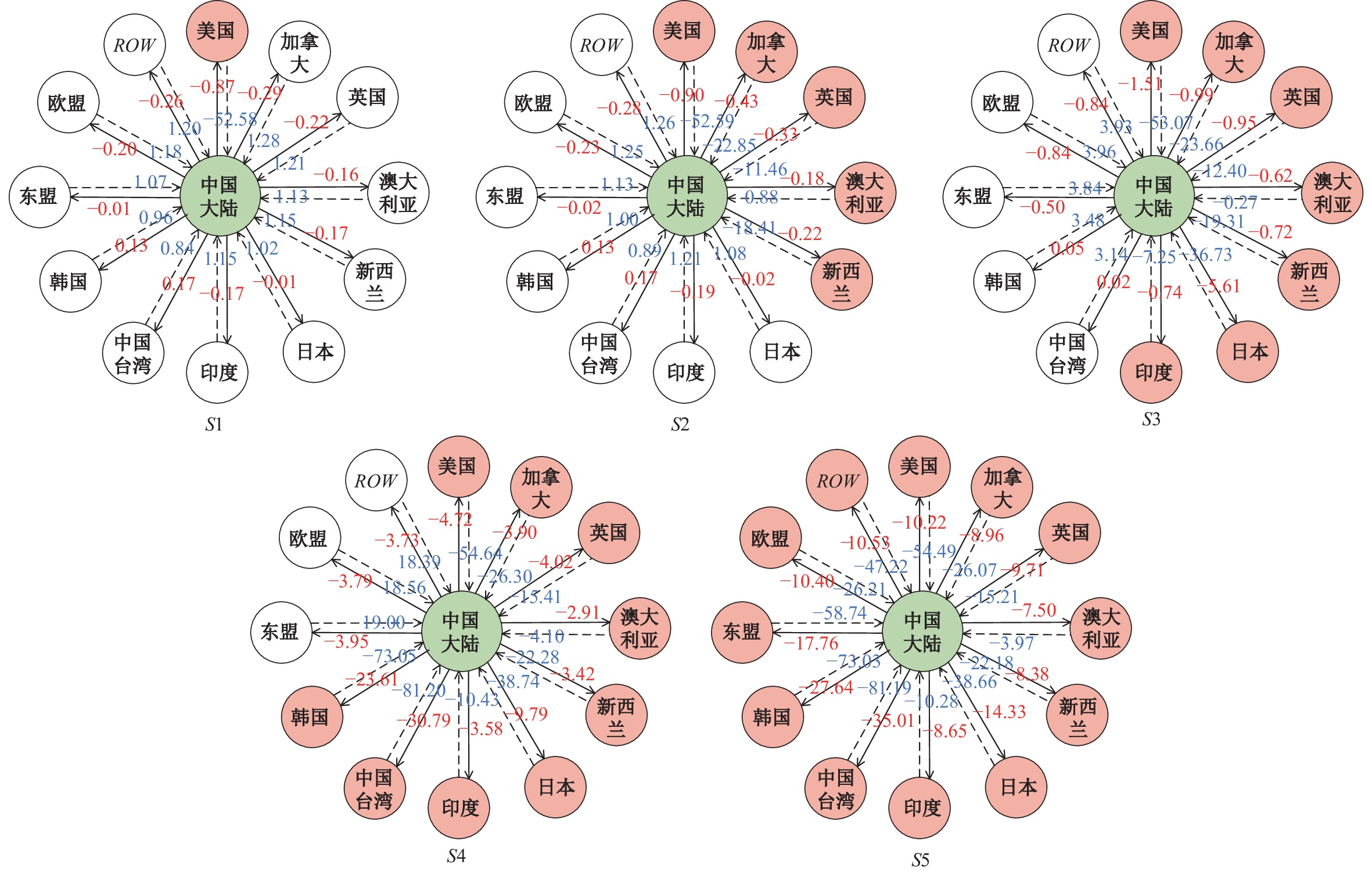﻿ 国际禁运联盟、供应链中断风险与我国宏观经济易损性《财经研究》
2022第48卷第12期

1. 安徽财经大学 统计与应用数学学院，安徽 蚌埠 233000;
2. 中国科学院 科技战略咨询研究院，北京 100190

Embargo Alliance, Supply Chain Disruption and China’s Macroeconomic Vulnerability: Taking Chips as an Example
Cui Lianbiao1, Weng Shimei1, Mo Jianlei2, Song Malin1, Xia Yan2
1. School of Statistics and Applied Mathematics, Anhui University of Finance & Economics, Bengbu 233000, China;
2. Institute of Science and Development, Chinese Academy of Sciences, Beijing 100190, China
Summary: The United States is trying to build up an alliance to continuously strengthen the technological blockade against China. Chips, as the core carrier of high technology, constitute the main battlefield in the Sino-US competition. Under the context of an embargo alliance forged by the United States against China and the risk of chip supply chain disruption confronting the world’s second largest economy, it is of great significance to quantitatively evaluate the vulnerability of China’s macro-economy and its impact on the border of chip import disruption. This paper develops a dynamic computable general equilibrium model consisting of 13 regions and 12 sectors around the world to make a simulation study of this issue. Simulation results show that the implementation of chip embargo imposed by the United States alone tends to have a limited impact on the Chinese economy, but when Taiwan Province of China and South Korea join the chip embargo alliance, the Chinese economy will suffer huge losses. Under the extreme scenario of complete interruption of chip imports, China’s real GDP will decrease by about 0.49%, with exports and employment declining by 8.02% and 0.18%, respectively. Worse still, the number of unemployed people will soar by about 1.36 million. Due to the trade transfer effect, there is significant heterogeneity in the economic impact of chip embargo alliance for countries and regions inside and outside the alliance. Specifically, members of the alliance will lose, while its non-members will benefit from the US-led chip embargo alliance. Moreover, the economic impact of the chip embargo alliance on its members is also asymmetric. Specifically, if Taiwan Province of China and South Korea join the US-led chip embargo alliance, their economies will sustain heavier losses than the United States. In addition, this paper also finds that China’s subsidy policy for the chip industry may help to alleviate the negative impact of the US chip embargo on the Chinese economy. This paper makes the following contributions: First, it is the first-ever one that evaluates the macroeconomic impact of the US-led chip embargo on China from the perspective of the alliance of major powers. This paper analyzes the evolution trend and law of the embargo in time dimension, revealing the impact boundary of chip supply and the pressure of China’s economic system. Second, it is the first study that analyzes the regional spillover effect of the chip embargo from the perspective of space trade transfer. Furthermore, this paper also estimates the trade transfer effect between relevant countries/regions and China under different scenarios and corresponding benefits and losses. Third, it introduces the concept of “virtual tariff cost” into the international trade model, and quantitatively depicts the interruption of chip imports caused by the intervention of national administrative forces, which better captures the non-market behavior in the chip embargo.
Key words: chip trade    embargo alliance    supply chain disruption    economic vulnerability    dynamic computable general equilibrium model

（一）全球芯片贸易变化趋势

（二）中国大陆芯片进口来源分析图 1 中国芯片进口变化趋势图 2 中国大陆芯片进口来源国或地区

（一）芯片禁运对居民福利的影响机理图 3 芯片进口受阻对居民福利的影响机理

（二）模型方法

GDYN模型假设行业产出有本地消费和出口两个去向，在常转换替代弹性（Constant Elasticity of Transformation，CET）的假定下，销量的分配满足企业利润最大化目标。对于进口贸易，GDYN模型假定进口品与本地生产品是非同质的，两者之间不能完全替代，且不同进口来源地的产品也不能完全替代，它们均满足阿明顿假设。对于进口关税，GDYN模型假设存在两类关税变量，即对所有贸易伙伴同时实施的关税壁垒和仅对某一国实施的特别关税壁垒，二者分别用变量 $TM$ 和变量 $TMS$ 表示。具体关系如式（1）所示：

 $PM{S_{i,r,s}} = PCI{F_{i,r,s}} \times \left( {1 + T{M_{i,s}}} \right) \times \left( {1 + TM{S_{i,r,s}}} \right)$ (1)

 $PM{S_{i,r,s}} = PCI{F_{i,r,s}} \times \left( {1 + T{M_{i,s}}} \right) \times \left( {1 + TM{S_{i,r,s}}} \right) \times \left( {1 + TMS{1_{i,r,s}}} \right)$ (2)

（三）模型校准与数据处理

 芯片进口额 （亿美元） 电子信息产品进口额 （亿美元） 占比 （%） 美国 195.51 377.52 51.79 加拿大 2.39 11.58 20.64 英国 2.58 37.37 6.90 澳大利亚 0.02 3.07 0.54 新西兰 0.07 0.59 11.69 日本 279.87 832.26 33.63 印度 0.09 16.35 0.56 中国台湾 1 267.15 1 588.93 79.75 韩国 747.20 1 060.83 70.43 东盟 722.44 1 333.35 54.18 欧盟 132.46 766.74 17.28 ROW 476.21 1 378.48 34.55 世界 3 825.98 7407.07 51.65

（四）芯片禁运联盟情景设置

（一）宏观经济影响

1. 实际GDP变化。表2展示了以无芯片禁运为参考基准，不同芯片禁运情景下各地区GDP的变化情况。随着芯片禁运联盟范围的不断扩大，中国大陆芯片进口短缺越发凸显，由此造成的宏观经济损失将不断增大。在S1情景下，2023年中国大陆GDPBAU情景下降0.018%，而在S5情景下，该降幅增至0.489%。从时间趋势看，芯片禁运对我国宏观经济的负面影响呈现逐步减缓之势，这主要是由于芯片禁运会倒逼我国将更多资源投入芯片研发和生产，而自产芯片供给的增加将在一定程度上对进口芯片形成替代。实际上，芯片作为一种高新技术产品，其准入门槛较高，自产芯片取代进口芯片不是一蹴而就的事情，故芯片禁运对我国经济的负面影响或将持续较长时间。

 S1 S2 S3 S4 S5 2023 2030 2023 2030 2023 2030 2023 2030 2023 2030 中国大陆 −0.018 −0.010 −0.019 −0.011 −0.065 −0.048 −0.314 −0.234 −0.489 −0.389 美国 −0.054 −0.055 −0.054 −0.055 −0.051 −0.052 −0.055 −0.057 −0.041 −0.042 加拿大 −0.003 −0.002 −0.012 −0.012 −0.015 −0.015 −0.027 −0.026 −0.045 −0.046 英国 −0.002 −0.002 −0.009 −0.009 −0.010 −0.010 −0.018 −0.019 −0.012 −0.013 澳大利亚 −0.003 −0.003 −0.003 −0.003 0.007 0.007 0.031 0.031 0.033 0.033 新西兰 −0.002 −0.002 −0.004 −0.004 0.002 0.003 0.020 0.022 0.027 0.030 日本 0.008 0.008 0.008 0.009 −0.217 −0.217 −0.268 −0.267 −0.258 −0.257 印度 −0.001 −0.002 −0.001 −0.002 −0.003 −0.004 −0.013 −0.018 0.023 0.021 中国台湾 0.022 0.022 0.024 0.023 0.107 0.105 −1.463 −1.378 −1.466 −1.379 韩国 0.015 0.014 0.016 0.015 0.067 0.062 −1.054 −1.036 −1.075 −1.059 东盟 0.003 0.003 0.004 0.004 0.024 0.024 0.144 0.147 −0.290 −0.339 欧盟 0.000 0.000 0.000 0.000 0.002 0.002 0.006 0.005 −0.023 −0.025 ROW −0.002 −0.002 −0.002 −0.002 0.001 0.001 0.008 0.005 −0.016 −0.022 世界 −0.015 0.000 −0.015 0.000 −0.031 0.000 −0.104 0.000 −0.156 0.000

2. 居民福利变化。在GDYN模型中，居民福利采用希克斯等价变差（Hicks Equivalent VariationHEV）进行表征，该度量方式综合考虑了人均总效用和区域总收入的复合效应。表3列举了不同芯片禁运情景下各地区居民福利的变化情况。与芯片进口中断导致的经济增长放缓有关，我国居民福利也将遭受一定程度的损失，且随着芯片禁运范围的扩大，福利损失越显著。在S1情景下，2023年中国大陆居民福利水平较BAU情景损失33.31亿美元，而在S5情景下，该损失大幅攀升至871.09亿美元。从时间维度来看，与GDP指标变化类似，芯片禁运对我国居民福利的负面冲击有所减缓，这主要是因为国内芯片生产投入的不断增加部分对冲了进口芯片断供的冲击。

 S1 S2 S3 S4 S5 2023 2030 2023 2030 2023 2030 2023 2030 2023 2030 中国大陆 −33.31 −1.53 −34.74 −1.19 −116.85 −35.52 −536.48 −242.73 −871.09 −434.06 美国 −37.60 −79.44 −37.83 −40.00 −36.74 −81.46 −55.61 −111.27 −75.24 −170.40 加拿大 −0.29 −0.85 −0.90 −1.05 −1.11 −2.09 −1.76 −1.97 −7.81 −13.37 英国 −1.16 −1.75 −2.06 −1.71 −2.57 −4.09 −6.89 −9.59 −10.11 −20.15 澳大利亚 −0.39 −0.46 −0.41 −0.25 0.59 1.21 4.07 7.56 0.22 −2.57 新西兰 −0.05 −0.06 −0.07 −0.06 0.03 0.00 0.23 0.31 −0.04 −0.56 日本 1.97 2.33 2.08 1.21 −89.87 −114.29 −123.90 −148.86 −129.49 −159.42 印度 −0.41 −2.10 −0.42 −1.10 −0.83 −4.32 −3.31 −12.54 −2.66 −32.81 中国台湾 1.62 2.09 1.71 1.11 7.43 8.85 −116.56 −173.31 −119.25 −178.35 韩国 2.58 3.35 2.72 1.77 11.45 13.41 −186.92 −286.53 −192.63 −299.22 东盟 0.05 0.97 0.07 0.63 6.33 9.39 47.08 68.00 −79.96 −219.55 欧盟 −3.95 −6.41 −4.19 −3.43 −2.78 −6.11 −12.69 −20.96 −80.07 −152.62 ROW −4.23 −14.34 −4.42 −7.47 −0.04 −9.05 27.19 21.82 −64.80 −270.85 世界 −75.17 −98.20 −78.46 −51.54 −224.95 −224.08 −965.54 −910.06 −1 632.92 −1 953.93

3. 区域进出口变化。表4展示了芯片禁运对全球不同地区出口及进口的影响。首先，封锁导致的芯片短缺会增加我国主要行业的生产成本，从而削弱相关产品的国际竞争力，导致出口减少，且随着芯片禁运范围的扩大，我国出口贸易受损将增加。以S1情景为例，当美国单独对我国实施芯片出口禁运时，我国当年出口较BAU情景下降0.28%，这一降幅低于美国的0.61%。值得注意的是，美国单边实施芯片出口禁运将带来一定的贸易转移效应，未跟随美国实施芯片出口禁运的中国台湾、韩国、东盟和日本等地区对中国大陆的出口额将分别增加0.07%、0.06%、0.05%和0.04%。而在将中国台湾、韩国、日本和印度等地区纳入禁运联盟后（S4情景），中国大陆出口降幅进一步增至4.35%，在最极端情景（S5）下，中国大陆出口贸易额锐减8.02%。

 出口 进口 S1 S2 S3 S4 S5 S1 S2 S3 S4 S5 2023 2030 2023 2030 2023 2030 2023 2030 2023 2030 2023 2030 2023 2030 2023 2030 2023 2030 2023 2030 中国大陆 −0.28 −0.24 −0.29 −0.25 −1.01 −0.82 −4.35 −3.77 −8.02 −7.77 −0.33 −0.26 −0.34 −0.27 −1.11 −0.81 −4.67 −3.71 −8.78 −8.01 美国 −0.61 −0.62 −0.61 −0.62 −0.68 −0.68 −1.03 −0.93 −1.29 −1.10 −0.31 −0.31 −0.32 −0.31 −0.33 −0.31 −0.42 −0.38 −0.61 −0.56 加拿大 0.01 0.00 −0.04 −0.04 −0.09 −0.10 −0.23 −0.24 −0.25 −0.24 0.00 0.00 −0.04 −0.03 −0.06 −0.04 −0.08 −0.04 −0.13 −0.08 英国 0.01 0.02 −0.02 −0.02 −0.06 −0.06 −0.20 −0.17 −0.27 −0.20 −0.01 −0.01 −0.04 −0.03 −0.05 −0.04 −0.10 −0.08 −0.13 −0.12 澳大利亚 0.00 0.00 0.00 0.00 −0.04 −0.02 −0.16 −0.08 −0.25 −0.13 −0.02 0.00 −0.02 0.00 0.00 0.04 0.08 0.23 −0.06 0.10 新西兰 −0.00 −0.00 −0.02 −0.01 −0.05 −0.03 −0.10 −0.04 −0.16 −0.08 −0.01 −0.01 −0.03 −0.02 −0.02 0.00 −0.01 0.07 −0.08 −0.01 日本 0.04 0.05 0.04 0.05 −0.98 −0.98 −1.34 −1.27 −1.63 −1.48 0.04 0.04 0.04 0.04 −1.79 −1.75 −2.28 −2.22 −2.57 −2.50 印度 −0.02 −0.02 −0.02 −0.02 −0.09 −0.07 −0.35 −0.26 −0.44 −0.34 −0.02 −0.01 −0.02 −0.02 −0.05 −0.04 −0.13 −0.09 −0.22 −0.21 中国台湾 0.07 0.07 0.08 0.07 0.33 0.29 −5.17 −4.89 −5.42 −5.10 0.12 0.10 0.12 0.10 0.53 0.43 −8.20 −8.15 −8.53 −8.47 韩国 0.06 0.06 0.06 0.06 0.22 0.20 −3.90 −3.83 −4.18 −4.07 0.09 0.08 0.10 0.08 0.38 0.30 −5.80 −5.80 −6.07 −6.06 东盟 0.05 0.04 0.05 0.04 0.16 0.12 0.79 0.64 −2.31 −2.62 0.04 0.03 0.04 0.03 0.18 0.12 0.92 0.68 −2.24 −2.38 欧盟 0.01 0.01 0.01 0.01 0.01 0.00 −0.01 −0.01 −0.17 −0.15 0.00 0.00 0.00 0.00 0.01 0.01 0.05 0.03 −0.10 −0.13 ROW −0.00 −0.00 −0.00 −0.00 −0.01 −0.01 −0.06 −0.04 −0.25 −0.26 −0.01 −0.01 −0.01 −0.01 −0.01 0.00 0.05 0.06 −0.22 −0.29

4. 区域就业变化。芯片封锁不仅重创全球供应链，冲击各地区经济增长，还对多数地区的就业市场产生负面影响。表5展示了政策冲击初期（2023年）各地区就业的变化情况。可知，我国遭遇芯片禁运后，就业市场将会遭受一定程度的负面影响，且随着芯片出口禁运的升级，我国失业人数将不断增加。当只有美国对我国实施芯片封锁（S1）时，我国就业人数相较BAU情景减少8.20万人，就业下降0.01%；当“五眼联盟”对我国实施芯片禁运（S2）时，我国失业人数较BAU情景增加8.56万人；而所有地区均对我国实施芯片禁运（S5）时，我国就业人数较BAU下降0.18%，失业人数增加136.35万人，对就业市场的冲击较大。

 就业变化比例（相对BAU，%） 就业变化人数（相对BAU，万人） S1 S2 S3 S4 S5 S1 S2 S3 S4 S5 中国大陆 −0.01 −0.01 −0.04 −0.13 −0.18 −8.20 −8.56 −27.69 −104.01 −136.35 美国 −0.07 −0.07 −0.07 −0.07 −0.05 −11.60 −11.61 −11.01 −11.73 −8.20 加拿大 −0.00 −0.02 −0.03 −0.05 −0.07 −0.05 −0.37 −0.47 −0.84 −1.33 英国 −0.00 −0.01 −0.02 −0.03 −0.02 −0.06 −0.46 −0.53 −0.96 −0.52 澳大利亚 −0.00 0.00 0.01 0.04 0.05 −0.05 −0.05 0.12 0.53 0.65 新西兰 −0.00 −0.01 0.00 0.03 0.05 −0.01 −0.02 0.01 0.08 0.13 日本 0.02 0.02 −0.45 −0.54 −0.51 1.11 1.17 −29.80 −36.01 −34.16 印度 −0.00 0.00 −0.01 −0.02 0.03 −1.00 −1.03 −2.07 −9.04 10.43 中国台湾 0.04 0.04 0.19 −2.74 −2.74 0.48 0.50 2.19 −31.56 −31.61 韩国 0.03 0.03 0.11 −1.85 −1.87 0.75 0.79 3.01 −50.22 −50.85 东盟 0.01 0.01 0.05 0.27 −0.60 0.20 0.21 1.39 8.15 −18.18 欧盟 0.00 0.00 0.00 0.01 −0.04 0.11 0.11 0.41 1.21 −3.41 ROW 0.00 0.00 0.00 0.02 −0.03 −0.44 −0.44 0.51 2.39 −4.13

（二）行业层面影响

1. 全球电子信息行业产出变化。现行的国民经济核算体系和诸多CGE模型大多将芯片纳入到电子信息行业予以测度，美国主导的芯片出口禁运对不同地区电子信息行业的冲击不尽相同：一是由于进口替代效应的存在，中国大陆电子信息行业产出将会增加；二是对实施出口禁运的国家或地区而言，其电子信息行业的产出将下降；三是由于贸易转移效应，未跟随美国对我国实施出口禁运的国家或地区的电子信息行业产出可能增加。

 S1 S2 S3 S4 S5 2023 2030 2023 2030 2023 2030 2023 2030 2023 2030 中国大陆 0.36 0.46 0.37 0.47 1.20 1.34 5.11 5.92 9.45 10.85 美国 −1.80 −2.21 −1.79 −2.20 −1.56 −2.13 −0.94 −2.12 0.13 −1.05 加拿大 0.10 −0.01 −1.02 −1.34 −0.79 −1.34 −0.09 −1.54 1.13 −0.35 英国 0.20 0.11 −0.20 −0.36 0.03 −0.31 0.82 −0.21 1.85 0.92 澳大利亚 0.26 0.09 0.26 0.09 0.69 0.20 2.27 0.46 4.67 3.07 新西兰 0.22 0.08 −0.10 −0.29 0.36 −0.09 1.91 0.42 4.10 2.70 日本 0.43 0.32 0.46 0.34 −7.69 −9.17 −7.89 −10.43 −7.52 −10.04 印度 0.17 0.07 0.18 0.08 0.43 0.14 1.56 0.54 3.30 2.35 中国台湾 0.43 0.33 0.45 0.35 1.55 1.19 −27.07 −30.18 −27.65 −30.86 韩国 0.46 0.35 0.49 0.37 1.70 1.30 −22.57 −25.30 −22.75 −25.54 东盟 0.41 0.27 0.44 0.29 1.34 0.89 5.52 3.76 −13.73 −15.64 欧盟 0.21 0.12 0.22 0.13 0.63 0.37 2.28 1.37 0.76 −0.38 ROW 0.10 −0.02 0.10 −0.01 0.50 0.19 1.98 0.92 0.87 −0.91

2. 全球电子信息行业双边贸易变化情况。图4列举了不同芯片禁运情景下中国大陆与主要国家或地区间电子信息行业双边贸易的变化情况（以2023年为例）。芯片禁运会带来复杂的贸易转移效应：参与禁运联盟的国家或地区对中国大陆电子信息行业的出口明显减少，而未加入禁运联盟的国家或地区则会增加，这在一定程度上缓解了芯片短缺的负面冲击。在S1情景下，美国对中国大陆电子信息行业出口大幅下降52.58%，中国大陆对美出口会小幅下降0.87%，而英国和澳大利亚等对中国大陆电子信息产品出口将会增加。随着禁运的升级，这种贸易转移效应会更为明显。特别是在中国台湾、韩国、日本加入禁运联盟的情景（S4）下，三者对中国大陆的出口分别下降81.20%、73.05%和38.74%，而东盟和欧盟等地的出口均将增加19%左右。这种贸易转移效应为中国应对美国芯片封锁赢得了一定的空间，即中国可争取与美国以外地区加强合作，利用市场机制降低美国芯片出口禁运带来的负面冲击。图 4 芯片禁运情景下中国大陆与主要国家或地区电子信息行业贸易变化（%）

3. 对我国分行业产出的影响。表7列举了不同芯片禁运情形下我国分行业产出的变化情况。可以看出，芯片封锁对我国不同行业的影响具有异质性，除了芯片所属的电子信息行业以外，其他行业产出较BAU情景均呈现不同程度的下降。其中，对芯片需求较大的电器设备行业所遭受的负面冲击最大，其产出降幅将由S1情景的0.06%增至S5情景的1.96%，该结果与电器设备行业生产对芯片投入的依赖性较强有关。根据GDYN 10数据库测算，中国电子信息行业对电器设备行业的中间投入系数为8.31%，而其对农林业、牧渔业、采掘业、食品烟草、纺织皮革和重工业的中间投入系数均不足1%。相对来看，重工业和服务业所受负面影响较小，其产出降幅分别由S1的0.00%和0.02%增至S5的0.16%和0.35%。

 S1 S2 S3 S4 S5 2023 2030 2023 2030 2023 2030 2023 2030 2023 2030 农林业 −0.03 −0.04 −0.03 −0.04 −0.08 −0.10 −0.31 −0.39 −0.65 −0.81 牧渔业 −0.03 −0.03 −0.03 −0.03 −0.08 −0.09 −0.32 −0.35 −0.60 −0.67 采掘业 −0.03 −0.05 −0.04 −0.05 −0.10 −0.13 −0.33 −0.47 −0.93 −1.19 食品烟草 −0.02 −0.02 −0.02 −0.02 −0.06 −0.06 −0.25 −0.24 −0.48 −0.47 纺织皮革 −0.06 −0.09 −0.06 −0.09 −0.19 −0.24 −0.91 −1.10 −1.68 −1.99 轻工业 −0.05 −0.06 −0.05 −0.06 −0.16 −0.16 −0.60 −0.63 −1.11 −1.19 重工业 0.00 0.00 0.00 0.00 −0.02 −0.02 −0.12 −0.09 −0.16 −0.14 电子信息行业 0.36 0.46 0.37 0.47 1.20 1.34 5.11 5.92 9.45 10.85 电器设备 −0.06 −0.07 −0.07 −0.07 −0.33 −0.29 −1.24 −1.18 −1.96 −1.95 运输设备 −0.05 −0.05 −0.05 −0.05 −0.20 −0.19 −0.69 −0.66 −1.14 −1.14 邮电行业 −0.02 −0.01 −0.02 −0.01 −0.05 −0.02 −0.22 −0.09 −0.36 −0.19 服务业 −0.02 −0.01 −0.02 −0.01 −0.05 −0.03 −0.21 −0.12 −0.35 −0.22

4. 对我国分行业进出口的影响。表8列举了不同禁运情景下我国各行业进出口贸易的变化情况（以2023年为例）。从出口来看，我国所有行业出口较BAU情景均出现下降，其中，电子信息行业降幅最为明显，其次是电器设备行业。以S4情景为例，上述两个行业出口分别下降8.48%和4.12%。从进口变化来看，除了电子信息行业，我国几乎所有行业进口均将增加。这是因为，芯片进口短缺会增加我国制造业的生产成本，削弱了自产品的竞争优势，从而导致进口增加。从变化幅度来看，电器设备进口增幅最为明显，这说明芯片进口中断对我国电器设备行业冲击最大，为弥补自产品供给不足，我国需要加大对同类产品的进口。换言之，美国主导的芯片封锁导致了复杂的行业溢出效应，间接导致我国部分高端制造业在本地和国际两个市场的同步收缩。

 出口变化（相对BAU，%） 进口变化（相对BAU，%） S1 S2 S3 S4 S5 S1 S2 S3 S4 S5 农林业 −0.12 −0.13 −0.32 −0.82 −2.22 0.05 0.06 0.11 0.28 0.93 牧渔业 −0.16 −0.17 −0.55 −1.66 −3.68 0.05 0.06 0.10 0.29 0.97 采掘业 −0.25 −0.26 −0.44 −0.26 −4.49 0.07 0.08 0.15 0.36 1.65 食品烟草 −0.10 −0.11 −0.38 −1.23 −2.72 0.03 0.03 0.08 0.33 1.04 纺织皮革 −0.12 −0.13 −0.33 −1.49 −2.95 0.00 0.00 0.18 2.22 3.00 轻工业 −0.22 −0.23 −0.64 −2.39 −4.69 0.08 0.09 0.30 1.27 2.46 重工业 −0.13 −0.14 −0.44 −1.88 −3.90 0.06 0.07 0.33 1.86 3.09 电子信息行业 −0.48 −0.51 −1.87 −8.48 −15.29 −1.80 −1.89 −6.41 −27.26 −51.19 电器设备 −0.27 −0.28 −1.13 −4.12 −7.07 0.07 0.07 0.79 3.52 5.12 运输设备 −0.17 −0.18 −0.75 −2.53 −4.36 0.03 0.03 0.33 0.98 1.58 邮电行业 −0.17 −0.18 −0.49 −2.24 −4.21 0.07 0.07 0.19 0.81 1.70 服务业 −0.11 −0.12 −0.35 −1.40 −2.72 0.05 0.05 0.16 0.60 1.29

（三）中国应对策略研究

 S4 S4+5% S4+10% S4+20% S4+30% 2023 2030 2023 2030 2023 2030 2023 2030 2023 2030 实际GDP（%） −0.31 −0.23 −0.28 −0.20 −0.26 −0.17 −0.20 −0.11 −0.14 −0.05 居民福利（亿美元） −574.26 −242.73 −570.43 −209.03 −566.74 −175.81 −559.81 −108.71 −553.50 −42.81 出口（%） −4.34 −3.77 −4.26 −3.67 −4.17 −3.57 −3.99 −3.38 −3.81 −3.18 进口（%） −4.67 −3.71 −4.61 −3.65 −4.56 −3.63 −4.45 −3.54 −4.34 −3.45 电子信息行业产出（%） 5.11 5.92 5.48 6.29 5.85 6.65 6.59 7.38 7.33 8.12

① 如无特别说明，下文中我国的相关数据及分析均不包含港澳台地区。

  包群, 张志强. 地震的余波: 价值链断裂、进口停滞与贸易危机传染[J]. 经济学（季刊）, 2021(2): 577–596.  陈峰. 美国扩大出口管制实体清单对中国科技的影响和对策[J]. 情报杂志, 2022(8): 1–7. DOI:10.3969/j.issn.1002-1965.2022.08.001  李巍. 从接触到竞争: 美国对华经济战略的转型[J]. 外交评论, 2019(5): 54–80.  李真, 李茂林, 陈天明. 中国制造业的中间品依赖与出口贸易——基于中美贸易摩擦历史背景的分析[J]. 财经科学, 2021(6): 67–80. DOI:10.3969/j.issn.1000-8306.2021.06.007  李峥. 美国推动中美科技“脱钩”的深层动因及长期趋势[J]. 现代国际关系, 2020(1): 33–40.  裴长洪, 刘斌. 中国对外贸易的动能转换与国际竞争新优势的形成[J]. 经济研究, 2019(5): 4–15.  彭爽. 出口管制: 理论与政策[M]. 北京: 经济科学出版社, 2018.  吴晓波, 张馨月, 沈华杰. 商业模式创新视角下我国半导体产业“突围”之路[J]. 管理世界, 2021(3): 123–136. DOI:10.3969/j.issn.1002-5502.2021.03.009  余典范, 王佳希, 张家才. 出口管制对中国企业创新的影响研究——以美国对华实体清单为例[J]. 经济学动态, 2022(2): 51–67.  于阳, 韩玉雄, 李怀祖. 出口管制政策能保持美国的技术领先优势吗?[J]. 世界经济, 2006(4): 42–48.  余振, 周冰惠, 谢旭斌, 等. 参与全球价值链重构与中美贸易摩擦[J]. 中国工业经济, 2018(7): 24–42.  朱启荣, 王玉平. 特朗普政府强化对中国技术出口管制的经济影响——基于“全球贸易分析模型”的评估[J]. 东北亚论坛, 2020(1): 54–68.  Costantini V, Sforna G. A dynamic CGE model for jointly accounting ageing population, automation and environmental tax reform. European Union as a case study[J]. Economic Modelling, 2020, 87: 280–306. DOI:10.1016/j.econmod.2019.08.004  Lakatos C, Walmsley T. Investment creation and diversion effects of the ASEAN-China free trade agreement[J]. Economic Modelling, 2012, 29(3): 766–779. DOI:10.1016/j.econmod.2012.02.004